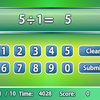# Arithmetic game

Fancy some interesting arithmetic training? Come and search for the numeric components and complete the equations in this game! You will be given a large grid of numerous numbers, while a questions will be presented at the bottom of the screen. Click to choose the correct numbers on the grid to complete the equation, then click the Submit button to check if the equation is valid. If the equation is correct, the numbers you picked will be removed from the grid, and you can proceed to the next question. If the formula is wrong, 5000 points will be deducted, and you need to click the Reset button above the equation to fill in the blanks again. The time you have spent will be recorded at the top of the screen. Can you complete all 7 questions in lightning speed to earn great rewards?

## Related software (5)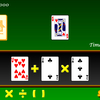## Make 24

Use the given cards to form a valid equation within 60 seconds!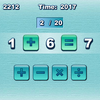## Quick Math

How quickly can you complete the equations with arithmetic symbols?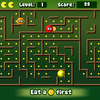## Math Man

Play Ghost Man with a mathematical twist!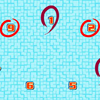## Make 15

Pick 3 numbers that add up to 15.